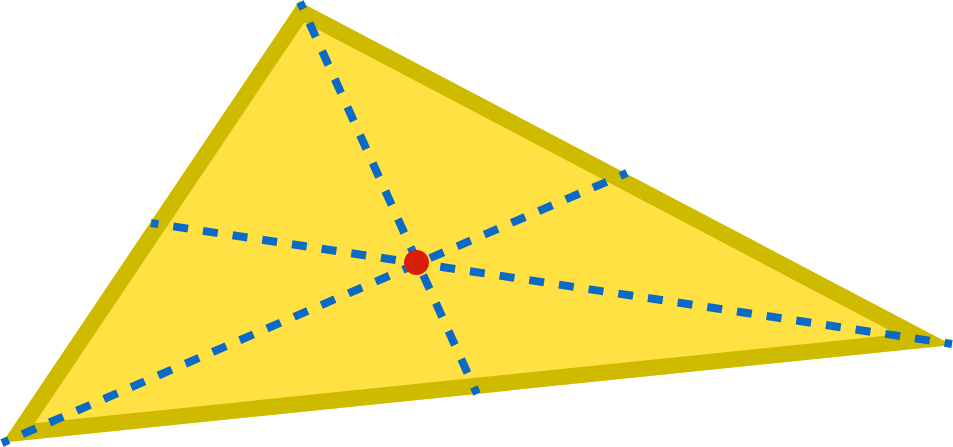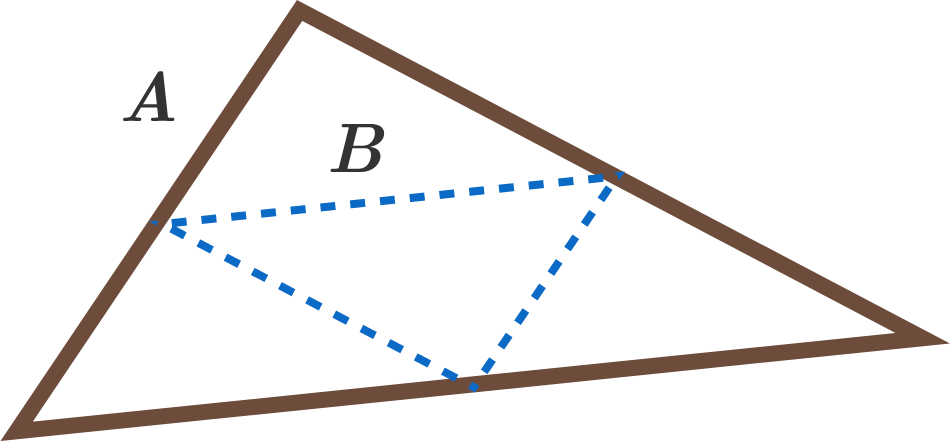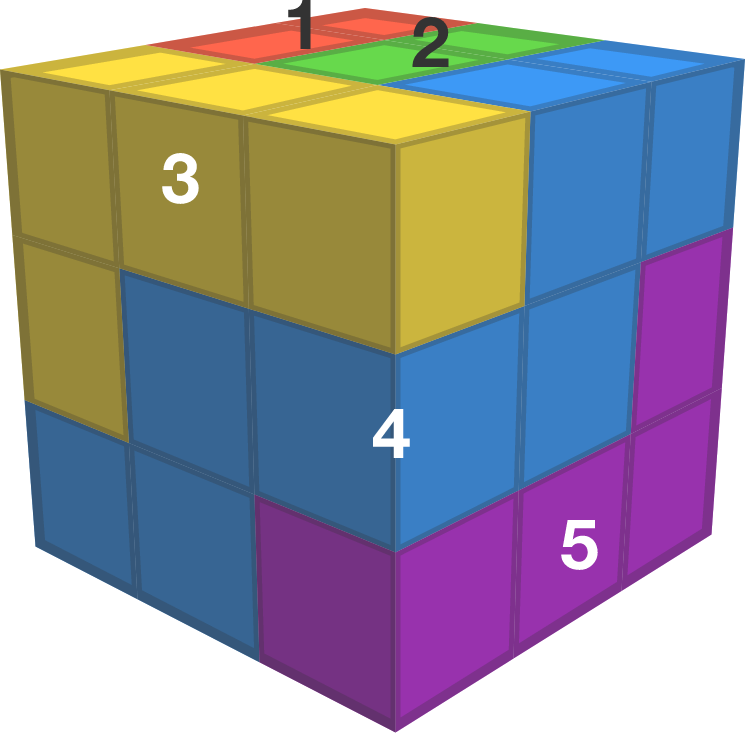# Problems of the Week

Contribute a problem

Let $N$ be the smallest positive integer for which $\frac{1}{1}+ \frac{1}{2} + \frac{1}{3} + \cdots + \frac{1}{N} > 100.$ How many digits does $N$ have?


Note: $\log_{10}e \approx 0.434.$

Andrea and Raleigh are each rolling a fair, six-sided die. They roll their dice simultaneously, individually keeping a sum until someone reaches 100; whoever reaches 100 first wins. (If they reach 100 on the same roll, it's a tie.)

Andrea's die has sides 1, 2, 3, 4, 5, and 6. Raleigh's has sides 1, 1, 1, 6, 6, and 6. Who is more likely to win?

The center of gravity of a solid triangle is the point where its medians intersect (its centroid).Consider a hollow triangle, $A,$ whose sides are made of uniformly dense, thin strings. Let $B$ be the triangle formed by connecting the midpoints of $A.$Where is the center of gravity of $A?$

For reference, here are the other three common triangle centers: circumcenter, incenter, and orthocenter.

Let $P(x)$ a polynomial in $x$ with real coefficients such that for all real numbers $x, y, z$ satisfying $xy+yz+zx=1,$ $P(x)+P(y)+P(z)=P(x+y+z).$ Furthermore, $P(0)=1$ and $P(1)=4.$ What is the value of $\sqrt{P(2017)}?$

An NEU path (North, East, and Up path) in 3-D is a sequence of cubes, where each successive cube is either 1 unit north, east, or up. When represented as lattice points in $\mathbb{Z}^3$, the steps have size $(1,0,0), (0,1,0)$ or $(0,0,1)$.

The goal is to tile a $27 \times 27 \times 27$ cube using NEU paths which fit entirely within the cube and do not overlap each other. What is the fewest number of NEU paths that are needed?An NEU path tiling of a $3 \times 3 \times 3$ cube with 7 paths (paths 6 and 7 are hidden from view)

As an explicit example, the minimal tiling of a $3 \times 3 \times 3$ cube with NEU paths requires 7 paths. An example is shown to the right, but 2 paths are hidden from view.

×